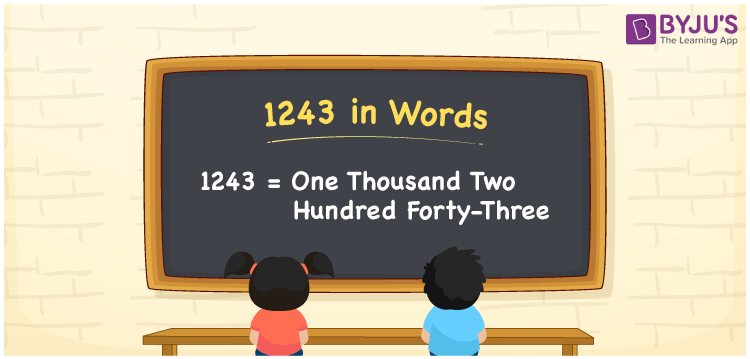# 1243 in Words

1243 in words can be written as One Thousand Two Hundred Forty-Three. If you spend Rs. 1243 to buy leather shoes, then you can say that “I spent One Thousand Two Hundred Forty-Three Rupees to buy leather shoes”. The numbers in words concept is very important to write numbers in the English alphabet based on the needs. Therefore, 1243 can be read as “One Thousand Two Hundred Forty-Three” in words.

 1243 in words One Thousand Two Hundred Forty-Three One Thousand Two Hundred Forty-Three in Numbers 1243

## 1243 in English Words## How to Write 1243 in Words?

Understand the place value of each digit in 1243 to write the numbers in English words. The place value chart is provided below for your reference.

 Thousands Hundreds Tens Ones 1 2 4 3

1243 in expanded form is:

1 x Thousand + 2 × Hundred + 4 × Ten + 3 × One

= 1 x 1000 + 2 × 100 + 4 × 10 + 3 × 1

= 1000 + 200 + 40 + 3

= 1243

= One Thousand Two Hundred Forty-Three

Therefore, 1243 in words is written as One Thousand Two Hundred Forty-Three.

1243 is a natural number that precedes 1244 and succeeds 1242.

1243 in words – One Thousand Two Hundred Forty-Three

Is 1243 an odd number? – Yes

Is 1243 an even number? – No

Is 1243 a perfect square number? – No

Is 1243 a perfect cube number? – No

Is 1243 a prime number? – No

Is 1243 a composite number? – Yes

## Frequently Asked Questions on 1243 in Words

Q1

### Write 1243 in words.

1243 can be written in words as “One Thousand Two Hundred Forty-Three”.
Q2

### How do you write One Thousand Two Hundred Forty-Three in numbers?

One Thousand Two Hundred Forty-Three can be written in numbers as 1243.
Q3

### Is 1243 an even number?

No, 1243 is not an even number as it is not completely divisible by 2.